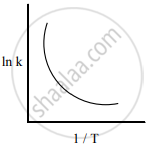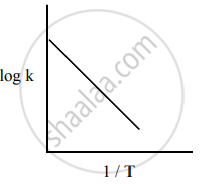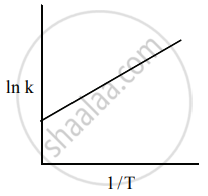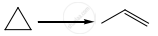Tamil Nadu Board of Secondary EducationHSC Science Class 12

# Tamil Nadu Board Samacheer Kalvi solutions for Class 12th Chemistry Volume 1 and 2 Answers Guide chapter 7 - Chemical Kinetics [Latest edition]

#### Chapters## Solutions for Chapter 7: Chemical Kinetics

Below listed, you can find solutions for Chapter 7 of Tamil Nadu Board of Secondary Education Tamil Nadu Board Samacheer Kalvi for Class 12th Chemistry Volume 1 and 2 Answers Guide.

Evaluation
Evaluation [Pages 226 - 231]

### Tamil Nadu Board Samacheer Kalvi solutions for Class 12th Chemistry Volume 1 and 2 Answers Guide Chapter 7 Chemical Kinetics Evaluation [Pages 226 - 231]

Evaluation | Q 1. | Page 226

For a first-order reaction $\ce{A -> B}$ the rate constant is x min−1. If the initial concentration of A is 0.01 M, the concentration of A after one hour is given by the expression.

• 0.01 e−x

• 1 × 10−2 (1 − e−60x)

• (1 × 10−2) e−60x

• none of these

Evaluation | Q 2. | Page 226

A zero-order reaction $\ce{X -> Product}$, with an initial concentration 0.02 M has a half-life of 10 min. if one starts with concentration 0.04 M, then the half-life is

• 10 s

• 5 min

• 20 min

• cannot be predicted using the given information

Evaluation | Q 3. | Page 226

Among the following graphs showing the variation of rate constant with temperature (T) for a reaction, the one that exhibits Arrhenius behavior over the entire temperature range is

•••• BothandEvaluation | Q 4. | Page 226

For a first order reaction $\ce{A ->Product}$ with initial concentration x mol L−1, has a half life period of 2.5 hours. For the same reaction with initial concentration ("x"/2) mol L−1 the half life is

• (2.5 × 2) hours

• (2.5/2) hours

• 2.5 hours

• Without knowing the rate constant, t1/2 cannot be determined from the given data

Evaluation | Q 5. | Page 227

For the reaction, $\ce{2NH3 -> N2 + 3H2}$, if (-"d" ["NH"_3])/"dt" = k1 [NH3], ("d" ["N"_2])/"dt" = k2 [NH3], ("d" ["H"_2])/"dt" = k3 [NH3] then the relation between k1, k2 and k3 is

• k1 = k2 = k3

• k1 = 3 k2 = 2 k3

• 1.5 k1 = 3 k2 = k3

• 2 k1 = k2 = 3 k3

Evaluation | Q 6. | Page 227

The decomposition of phosphine (PH3) on tungsten at low pressure is a first-order reaction. It is because the

• rate is proportional to the surface coverage

• rate is inversely proportional to the surface coverage

• rate is independent of the surface coverage

• rate of decomposition is slow

Evaluation | Q 7. | Page 227

For a reaction Rate = "k" ["acetone"]^(3/2) then unit of rate constant and rate of reaction respectively is

• ("mol L"^-1 "s"^-1), ("mol"^((-1)/2) "L"^(1/2) "s"^-1)

• ("mol"^((-1)/2) "L"^(1/2) "s"^-1), ("mol L"^-1 "s"^-1)

• ("mol"^(1/2) "L"^(1/2) "s"^-1), ("mol L"^-1 "s"^-1)

• ("mol L s"^-1), ("mol"^(1/2) "L"^(1/2) "s")

Evaluation | Q 8. | Page 227

The addition of a catalyst during a chemical reaction alters which of the following quantities?

• Enthalpy

• Activation energy

• Entropy

• Internal energy

Evaluation | Q 9. | Page 227

Consider the following statements:

(i) increase in concentration of the reactant increases the rate of a zero-order reaction.

(ii) rate constant k is equal to collision frequency A if Ea = 0

(iii) rate constant k is equal to collision frequency A if Ea = ∞

(iv) a plot of ln (k) vs T is a straight line.

(v) a plot of ln (k) vs (1/"T") is a straight line with a positive slope.

Correct statements are

• (ii) only

• (ii) and (iv)

• (ii) and (v)

• (i), (ii) and (v)

Evaluation | Q 10. | Page 227

In a reversible reaction, the enthalpy change and the activation energy in the forward direction are respectively −x kJ mol−1 and y kJ mol−1. Therefore , the energy of activation in the backward direction is

• (y − x) kJ mol−1

• (x + y) J mol−1

• (x − y) kJ mol−1

• (x + y) × 103 J mol−1

Evaluation | Q 11. | Page 228

What is the activation energy for a reaction if its rate doubles when the temperature is raised from 200 K to 400 K? (R = 8.314 JK−1mol−1)

• 234.65 kJ mol1

• 434.65 kJ mol−1

• 2.305 kJ mol1

• 334.65 J mol1

Evaluation | Q 12. | Page 228This reaction follows first-order kinetics. The rate constant at particular temperature is 2.303 × 10−2 hour−1. The initial concentration of cyclopropane is 0.25 M. What will be the concentration of cyclopropane after 1806 minutes? (log 2 = 0.3010)

• 0.125 M

• 0.215 M

• 0.25 × 2.303 M

• 0.05 M

Evaluation | Q 13. | Page 228

For a first-order reaction, the rate constant is 6.909 min−1 the time taken for 75% conversion in minutes is

• (3/2) log 2

• (2/3) log 2

• (3/2) log (3/4)

• (2/3) log (4/3)

Evaluation | Q 14. | Page 228

In a first order reaction $\ce{x -> y}$; if k is the rate constant and the initial concentration of the reactant x is 0.1 M, then, the half life is

• ((log 2)/"k")

• (0.693/((0.1) "k"))

• ((ln 2)/"k")

• none of these

Evaluation | Q 15. | Page 228

Predict the rate law of the following reaction based on the data given below.

$\ce{2A + B -> C + 3D}$

 Reaction number [A](min) [B](min) Initial rate(M s−1) 1 0.1 0.1 x 2 0.2 0.1 2x 3 0.1 0.2 4x 4 0.2 0.2 8x
• rate = k [A]2 [B]

• rate = k [A] [B]2

• rate = k [A] [B]

• rate = "k" ["A"]^(1/2) ["B"]^(3/2)

Evaluation | Q 16. | Page 228

Assertion: rate of reaction doubles when the concentration of the reactant is doubles if it is a first-order reaction.

Reason: rate constant also doubles.

• Both assertion and reason are true and reason is the correct explanation of assertion.

• Both assertion and reason are true but reason is not the correct explanation of assertion.

• Assertion is true but reason is false.

• Both assertion and reason are false.

Evaluation | Q 17. | Page 228

The rate constant of a reaction is 5.8 × 10−2 s−1. The order of the reaction is ____________.

• First order

• Zero order

• Second order

• Third order

Evaluation | Q 18. | Page 228

For the reaction $\ce{N2O5_{(g)} -> 2NO2_{(g)} + 1/2O2_{(g)}}$, the value of rate of disappearance of N2O5 is given as 6.5 × 10−2 mol L−1s−1. The rate of formation of NO2 and O2 is given respectively as

• (3.25 × 102 mol L-1s1) and (1.3 × 102 mol L1s1)

• (1.3 × 102 mol L1s1) and (3.25 × 102 mol L1s1)

• (1.3 × 101 mol L1s1) and (3.25 × 102 mol L1s1)

• None of these

Evaluation | Q 19. | Page 229

During the decomposition of H2O2 to give dioxygen, 48 g O2 is formed per minute at certain point of time. The rate of formation of water at this point is

• 0.75 mol min−1

• 1.5 mol min−1

• 2.25 mol min−1

• 3.0 mol min−1

Evaluation | Q 20. | Page 229

If the initial concentration of the reactant is doubled, the time for half reaction is also doubled. Then the order of the reaction is ____________.

• Zero

• One

• Fraction

• None

Evaluation | Q 21. | Page 229

In a homogeneous reaction $\ce{A -> B + C + D}$, the initial pressure was P0 and after time t it was P. Expression for rate constant in terms of P0, P and t will be

• "k" = (2.303/"t") log ((2"P"_0)/(3"P"_0 - "P"))

• "k" = (2.303/"t") log ((2"P"_0)/("P"_0 - "P"))

• "k" = (2.303/"t") log ((3"P"_0 - "P")/(2"P"_0))

• "k" = (2.303/"t") log ((2"P"_0)/(3"P"_0 - 2"P"))

Evaluation | Q 22. | Page 229

If 75% of a first order reaction was completed in 60 minutes, 50% of the same reaction under the same conditions would be completed in ____________.

• 20 minutes

• 30 minutes

• 35 minutes

• 75 minutes

Evaluation | Q 23. | Page 229

The half life period of a radioactive element is 140 days. After 560 days, 1 g of element will be reduced to

• (1/2) "g"

• (1/4) "g"

• (1/8) "g"

• (1/16) "g"

Evaluation | Q 24. | Page 229

The correct difference between first and second order reactions is that

• A first order reaction can be catalysed; a second order reaction cannot be catalysed.

• The half life of a first order reaction does not depend on [A0]; the half life of a second order reaction does depend on [A0].

• The rate of a first order reaction does not depend on reactant concentrations; the rate of a second order reaction does depend on reactant concentrations.

• The rate of a first order reaction does depend on reactant concentrations; the rate of a second order reaction does not depend on reactant concentrations.

Evaluation | Q 25. | Page 229

After 2 hours, a radioactive substance becomes (1/16)^"th" of original amount. Then the half life ( in min) is

• 60 minutes

• 120 minutes

• 30 minutes

• 15 minutes

Evaluation | Q 1. | Page 230

Define average rate.

Evaluation | Q 1. | Page 230

Define instantaneous rate.

Evaluation | Q 2. | Page 230

Define rate law.

Evaluation | Q 2. | Page 230

Define rate constant.

Evaluation | Q 3. | Page 230

Derive integrated rate law for a zero-order reaction $\ce{A -> Product}$.

Evaluation | Q 4. | Page 230

Define half life of a reaction.

Evaluation | Q 4. | Page 230

Show that for a first order reaction half life is independent of initial concentration.

Evaluation | Q 5. | Page 230

What is an elementary reaction?

Evaluation | Q 5. | Page 230

Give the differences between order and molecularity of a reaction.

Evaluation | Q 6. | Page 230

Explain the rate determining step with an example.

Evaluation | Q 7. | Page 230

Describe the graphical representation of first order reaction.

Evaluation | Q 8. (a) | Page 230

Write the rate law for the following reaction.

A reaction that is 3/2 order in x and zero order in y.

Evaluation | Q 8. (b) | Page 230

Write the rate law for the following reaction.

A reaction that is second order in NO and first order in Br2.

Evaluation | Q 9. | Page 230

Explain the effect of catalyst on reaction rate with an example.

Evaluation | Q 10. | Page 230

The rate law for a reaction of A, B and C has been found to be rate = "k" ["A"]^2["B"] ["L"]^(3/2). How would the rate of reaction change when

1. Concentration of [L] is quadrupled
2. Concentration of both [A] and [B] are doubled
3. Concentration of [A] is halved
4. Concentration of [A] is reduced to (1/3) and concentration of [L] is quadrupled.
Evaluation | Q 11. | Page 230

The rate of formation of a dimer in a second order reaction is 7.5 × 10−3 mol L−1s−1 at 0.05 mol L−1 monomer concentration. Calculate the rate constant.

Evaluation | Q 12. | Page 230

For a reaction $\ce{x + y + z -> products}$ the rate law is given by rate = "k" ["x"]^(3/2) ["y"]^(1/2) what is the overall order of the reaction and what is the order of the reaction with respect to z.

Evaluation | Q 13. | Page 230

Explain briefly the collision theory of bimolecular reactions.

Evaluation | Q 14. | Page 230

Write Arrhenius equation and explains the terms involved.

Evaluation | Q 15. | Page 230

The decomposition of Cl2O7 at 500 K in the gas phase to Cl2 and O2 is a first order reaction. After 1 minute at 500 K, the pressure of Cl2O7 falls from 0.08 to 0.04 atm. Calculate the rate constant in s−1.

Evaluation | Q 16. | Page 230

Give two examples for zero order reaction.

Evaluation | Q 17. | Page 230

Explain pseudo-first-order reaction with an example.

Evaluation | Q 18. (i) | Page 230

Identify the order for the following reaction.

Rusting of Iron

Evaluation | Q 18. (ii) | Page 230

Identify the order for the following reaction.

Evaluation | Q 18. (iii) | Page 230

Identify the order for the following reaction.

$\ce{2A + 3B -> products}$; rate = "k" ["A"]^(1/2) ["B"]^2

Evaluation | Q 19. | Page 230

A gas phase reaction has energy of activation 200 kJ mol−1. If the frequency factor of the reaction is 1.6 × 1013 s−1. Calculate the rate constant at 600 K. ("e"^-40.09 = 3.8 xx 10^-18)

Evaluation | Q 20. | Page 231

For the reaction $\ce{2x + y -> L}$ find the rate law from the following data.

 [x](M) [y](M) rate(M s−1) 0.2 0.02 0.15 0.4 0.02 0.30 0.4 0.08 1.20
Evaluation | Q 21. | Page 231

How do concentrations of the reactant influence the rate of reaction?

Evaluation | Q 22. | Page 231

How do nature of the reactant influence rate of reaction?

Evaluation | Q 23. | Page 231

The rate constant for a first order reaction is 1.54 × 10−3 s−1. Calculate its half life time.

Evaluation | Q 24. | Page 231

The half life of the homogeneous gaseous reaction $\ce{SO2Cl2 -> SO2 + Cl2}$ which obeys first order kinetics is 8.0 minutes. How long will it take for the concentration of SO2Cl2 to be reduced to 1% of the initial value?

Evaluation | Q 25. | Page 231

The time for half change in a first order decomposition of a substance A is 60 seconds. Calculate the rate constant. How much of A will be left after 180 seconds?

Evaluation | Q 26. | Page 231

A zero order reaction is 20% complete in 20 minutes. Calculate the value of the rate constant. In what time will the reaction be 80% complete?

Evaluation | Q 27. | Page 231

The activation energy of a reaction is 22.5 k Cal mol−1 and the value of rate constant at 40°C is 1.8 × 10−5 s−1. Calculate the frequency factor, A.

Evaluation | Q 28. | Page 231

Benzene diazonium chloride in aqueous solution decomposes according to the equation $\ce{C6H5N2Cl -> C6H5Cl + N2}$. Starting with an initial concentration of 10 g L−1, the volume of N2 gas obtained at 50°C at different intervals of time was found to be as under:

 t (min): 6 12 18 24 30 ∞ Vol. of N2(ml) 19.3 32.6 41.3 46.5 50.4 58.3

Show that the above reaction follows the first order kinetics. What is the value of the rate constant?

Evaluation | Q 29. | Page 231

From the following data, show that the decomposition of hydrogen peroxide is a reaction of the first order:

 t (min) 0 10 20 V (ml) 46.1 29.8 19.3

Where t is the time in minutes and V is the volume of standard KMnO4 solution required for titrating the same volume of the reaction mixture.

Evaluation | Q 30. | Page 231

A first order reaction is 40% complete in 50 minutes. Calculate the value of the rate constant. In what time will the reaction be 80% complete?

## Solutions for Chapter 7: Chemical Kinetics

Evaluation## Tamil Nadu Board Samacheer Kalvi solutions for Class 12th Chemistry Volume 1 and 2 Answers Guide chapter 7 - Chemical Kinetics

Shaalaa.com has the Tamil Nadu Board of Secondary Education Mathematics Class 12th Chemistry Volume 1 and 2 Answers Guide Tamil Nadu Board of Secondary Education solutions in a manner that help students grasp basic concepts better and faster. The detailed, step-by-step solutions will help you understand the concepts better and clarify any confusion. Tamil Nadu Board Samacheer Kalvi solutions for Mathematics Class 12th Chemistry Volume 1 and 2 Answers Guide Tamil Nadu Board of Secondary Education 7 (Chemical Kinetics) include all questions with answers and detailed explanations. This will clear students' doubts about questions and improve their application skills while preparing for board exams.

Further, we at Shaalaa.com provide such solutions so students can prepare for written exams. Tamil Nadu Board Samacheer Kalvi textbook solutions can be a core help for self-study and provide excellent self-help guidance for students.

Concepts covered in Class 12th Chemistry Volume 1 and 2 Answers Guide chapter 7 Chemical Kinetics are Integrated Rate Equations, First Order Reactions, Zero Order Reactions, Rate of a Chemical Reaction, Molecularity, Collision Theory, Arrhenius Equation – the Effect of Temperature on Reaction Rate, Factors Affecting the Reaction Rate, Pseudo First Order Reaction, Half Life Period of a Reaction.

Using Tamil Nadu Board Samacheer Kalvi Class 12th Chemistry Volume 1 and 2 Answers Guide solutions Chemical Kinetics exercise by students is an easy way to prepare for the exams, as they involve solutions arranged chapter-wise and also page-wise. The questions involved in Tamil Nadu Board Samacheer Kalvi Solutions are essential questions that can be asked in the final exam. Maximum Tamil Nadu Board of Secondary Education Class 12th Chemistry Volume 1 and 2 Answers Guide students prefer Tamil Nadu Board Samacheer Kalvi Textbook Solutions to score more in exams.

Get the free view of Chapter 7, Chemical Kinetics Class 12th Chemistry Volume 1 and 2 Answers Guide additional questions for Mathematics Class 12th Chemistry Volume 1 and 2 Answers Guide Tamil Nadu Board of Secondary Education, and you can use Shaalaa.com to keep it handy for your exam preparation.